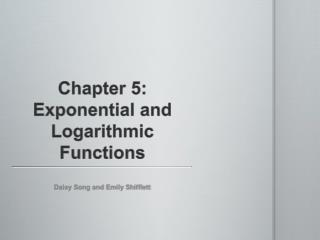DownloadDownload PresentationChapter 5: Exponential and Logarithmic Functions

# Chapter 5: Exponential and Logarithmic Functions

Download Presentation## Chapter 5: Exponential and Logarithmic Functions

- - - - - - - - - - - - - - - - - - - - - - - - - - - E N D - - - - - - - - - - - - - - - - - - - - - - - - - - -
##### Presentation Transcript

1. Chapter 5: Exponential and Logarithmic Functions Daisy Song and Emily Shifflett

2. Table of Contents 5.1: Composite Functions 5.2: One-to-One Functions; Inverse Functions 5.3: Exponential Functions 5.4: Logarithmic Functions 5.5: Properties of Logarithms 5.6: Logarithmic and Exponential Equations 5.7: Compound Interest 5.8: Exponential Growth and Decay Models; Newton’s Law; Logistic Growth and Decay Models 5.9: Building Exponential, Logarithmic, and Logistic Models from Data

3. 5.1: Composite Functions (f°g)(x)= f(g(x)) Domain (f °g) is all numbers in the domain of g(x) that coincide with domain of f(x).

4. 5.2: One-to-One Functions; Inverse Functions Function is one-to-one is x1 and x2 are two different inputs of a function and x1 ≠ x2 A function is one-to-one if, when graphed, a horizontal line ever only hits one point. A function that is increasing on an interval I is a one-to-one function on I. A function that is decreasing on an interval I is a one-to-one function on I.

5. 5.2 Continued • Inverse function of f: correspondence of the range of f back to the domain of f • Domain f= range f-1, range f= domain f-1 • x1=y1, x2=y2 y1=x1, y2=x2 where y1≠y2 and x1≠x2 • Verifying the Inverse: f(f-1(x))= x and f-1(f(x))=x • Finding the Inverse Function: x=Ay+B Ay+B=x Ay=x-B y= (x-B)/A

6. 5.3: Exponential Functions • f(x)=ax where a>0 and a≠1 • Laws of Exponents (as)(at)= as+t (as)t=ast (ab)s=asbs 1s=1 a-s=1/as= (1/a)s a0=1

7. 5.3 Continued • For f(x)=ax, a>0 and a≠1, if x is any real number then f(x+1)/f(x)=a • f(x)=ax+n • n moves the function up and down the y-axis • The number e: (1+(1/n))n=e • y=ex-n • n stretches the curve • If ab=ac then b=c

8. 5.4: Logarithmic Functions • y=logax if and only if x=ay • Domain: 0<x<∞ Range: -∞<x<∞ • y=lnx if and only if x=ey • Graphing Logarithmic Functions: • Plot y=ax of y=logax function • Draw the line y=x • Reflect graph

9. 5.4 Continued • Properties of Logarithmic Functions: • Domain is x>0, Range is all real numbers • x-intercept is 1. There is no y-intercept • The y-axis is a vertical asymptote • The function is decreasing if 0<a<1 and increasing if a>1 • The graph contains the points (1,0), (a,1), and (1/a, 1) • The graph is smooth and continuous, with no corners or gaps

10. 5.4 Continued • Graphing Inverse Logarithmic Functions: • Find domain of f(x) and graph • Find the range and vertical asymptote • Find f-1(x) • Use f-1 to find the range of f • Graph f-1

11. 5.5: Properties of Logarithms loga1=0 logaa=1 alogaM=M logaar=r loga(MN)= logaM+logaN loga(M/N)= logaM-logaN logaMr= rlogaM logaM= logbM/logba (a≠1, b≠1)

12. 5.6: Logarithm and Exponential Equations y=logax = x=ay if a>0, a≠1 If logaM=logaN then M=N if M,N, and a are positive and a≠1 If au=av then u=v if a>0, a≠1 Graphing calculator can be used to graph both sides of the equation and find the intersect

13. 5.7: Compound Interest • Simple Interest Formula: I=Prt • Interest=(amount borrowed)(interestrate)(years) • Payment periods • Annually: once per year • Semiannually: twice per year • Quarterly: four times per year • Monthly: 12 times per year • Daily: 365 times per year

14. 5.7 Continued • Compound Interest: Inew=Pold+Iold • Compound Interest Forumula: A=P(I+(r/n))nt • Amount= (Principal amount)(Interest+(rate/times per year))(number per year)(years) • Effective interest: annual simple rate of interest= compounding after one year • Present value: time value of money • P=A(1+(r/n)-nt if compound • P=Ae-rtif continuously compounded

15. 5.8: Exponential Growth and Decay; Newton’s Law; Logistic Growth and Decay • Law of Uninhibited Growth: A(t)=A0ekt A0=amount when t=0 k≠0 • Amount(time)= (original amount)e(constant)(time) • Uninhibited Radioactive Decay, and Uninhibited Growth of Cells follow this model • Half-life: time required for ½ of the substance to decay • Newton’s Law of Cooling: u(t)= T+(u0-T)ekt • Temperature(time)=constant temp+ (original temp-constant temp)e(constant)(time)

16. 5.8 Continued • Logistic Model: P(t)=c/1+ae-bt • Population(time)=carrying capacity/1+(constant)e -constant(time) • c=carrying capacity P(t) approaches c as t approaches ∞ • Describes situations where growth and decay is limited • b>0 growth rate, b<0 decay rate

17. 5.8 Continued • Properties of Logistic Growth Function: • Domain is all real numbers • Range 0<y<c • No x-intercepts, y-intercept at P(0) • Two horizontal asymptotes at y=o and y=c • P(t) is increasing if b>0 • P(t) is decreasing if b<0 • Inflection point= point where the graph changes direction (up to down for growth models and down to up for decay models)= P(t) ½ c • Smooth and continuous graph, no corners or gaps

18. 5.9: Building Exponential, Logarithmic, and Logistic Models from Data Same as finding line of best fit Draw a scatter diagram of the data to see the general shape to determine what type of function to use Many calculators have a Regression option to fit an equation to data To fit: enter data into calculator. Use correct regression to calculate model.